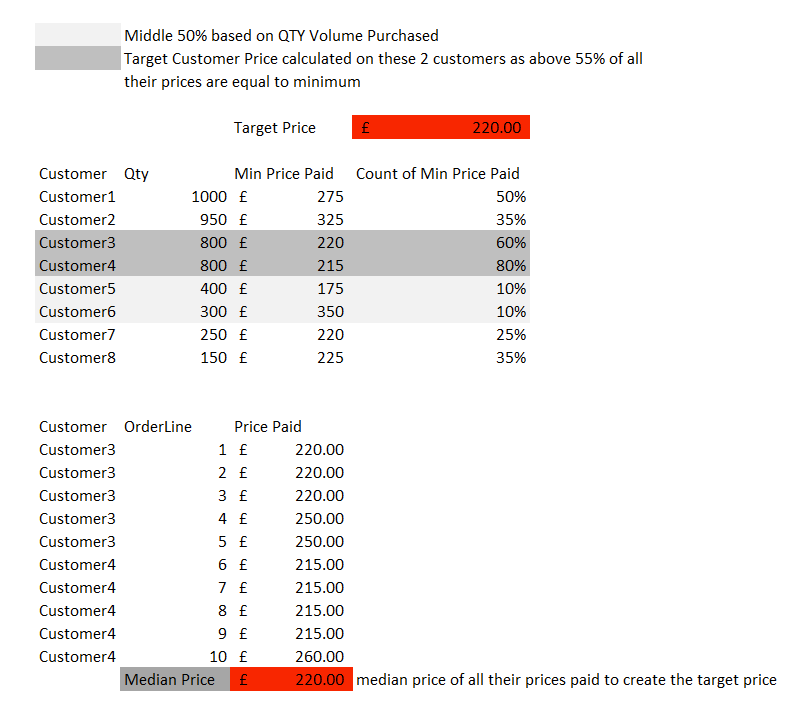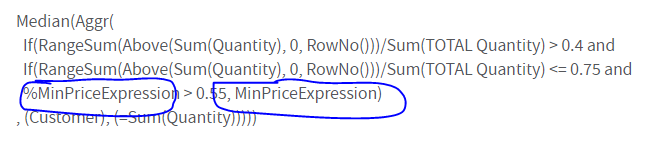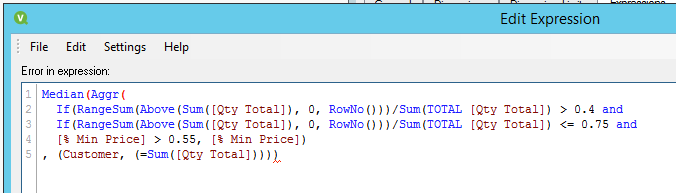# QlikView App Development

Discussion Board for collaboration related to QlikView App Development.

Announcements
Modernize Your QlikView Deployment webinar, Nov. 3rd. REGISTER
cancel
Showing results for
Did you mean:
HighlightedPartner

## Finding the middle 50% of range and median price paid

HI all,

looking for some help on a complex piece of logic that im struggling to find an answer for..

i am trying to find where the middle 50% range sits of the quantities purchased across all customers and of these middle 50% to then find where the count of min price paid is greater than 55% (previously answered by Sunny T with thanks), of their min prices paid and then from all these customers who fall into this logic (example shows customer 3,4 as qty in middle 50% and min price % 60,80%), then work out what target mode price would be for these 2 customers to create a realistic achievable price to be used for all other customers regardless of their price to calculate a guide price in which is achievable by all customers buying the same item.

i have attempted to draw it up in excel, the top table is already aggregated in a straight table to a customer level and the min price and count of min price logics working (thanks sunny). bottom table then shows the raw details which shows all prices paid by customer 3,4 of which we can work out a target price.i hope this is achievable , and if any further info is required, please feel free to ask or message and thanks in advance

Labels (4)

• ### QLIKVIEW Chart

4 Solutions

Accepted Solutions
HighlightedMVP

``````Median(Aggr(
If(
RangeSum(Above(Sum(Quantity), 0, RowNo()))/Sum(TOTAL Quantity) > 0.4 and
RangeSum(Above(Sum(Quantity), 0, RowNo()))/Sum(TOTAL Quantity) <= 0.75 and
%MinPriceExpression > 0.55
, MinPriceExpression)
, (Customer, (=Sum(Quantity)))))``````

Also, you replaced both the placeholders with %MinPrice... One if %MinPrice and other is MinPrice

HighlightedMVP

2 things

1) Sorting for ranking was changed...

2) Amount was not read as a number

Try attached

HighlightedMVP

I have updated the expression to

``````Median({<Customer>}TOTAL Aggr(
If(
RangeSum(Above(Sum({<Customer>}Quantity), 0, RowNo()))/Sum({<Customer>}TOTAL Quantity) > 0.4 and
RangeSum(Above(Sum({<Customer>}Quantity), 0, RowNo()))/Sum({<Customer>}TOTAL Quantity) <= 0.75 and
Min({<Customer>}%MinPriceExpression) > 0.55
, Only({<Customer>}MinPriceExpression))
, (Customer, (=Sum({<Customer>}Quantity), DESC)))) * Avg(1)``````
HighlightedMVP

Try this

``````min({<Customer>}TOTAL Aggr(
if(rank(sum({<Customer>}Quantity),4,1)	> fractile({<Customer>}TOTAL aggr (rank(sum({<Customer>}Quantity),4,1),Customer), 0.15) and
rank(sum({<Customer>}Quantity),4,1)	<= fractile({<Customer>}TOTAL aggr (rank(sum({<Customer>}Quantity),4,1),Customer), 0.70) and
Count({<Customer>}DISTINCT Aggr(If(Only({<Customer>} PricePaid) = Min({<Customer>}TOTAL <Customer> PricePaid), Only({<Customer>} [Order Line])), Customer, [Order Line]))/Count({<Customer>}DISTINCT [Order Line])>.55
, min({<Customer>}PricePaid))
, (Customer, (=Sum({<Customer>}Quantity), DESC)))) * Avg(1)``````
17 Replies
HighlightedMVP

May be share the Excel file so that we can take a look at the logic in Excel and try to implement it in Qlik

HighlightedPartner

the excel is only hard coded with the examples, i will see if i can update excel to be dynamic enough to calculate the values automatically and upload it to here

HighlightedPartner

HI Sunny, attached i sExcel doc, i am hoping helps with the logic...

ideally i was hoping to work out median price on all raw rows, but for ease on excel, im doing median of the 3 prices that are equal to the correct logic outcomes... i would like to set this in a text object as overall achievable price and also a column across all customers as shown

i hope this helps to identify the requirement..

let me know if anything else is needed

HighlightedMVP

May be something like this

``````Median(Aggr(
If(RangeSum(Above(Sum(Quantity), 0, RowNo()))/Sum(TOTAL Quantity) > 0.4 and
If(RangeSum(Above(Sum(Quantity), 0, RowNo()))/Sum(TOTAL Quantity) <= 0.75 and
%MinPriceExpression > 0.55, MinPriceExpression)
, (Customer, (=Sum(Quantity))))``````

HighlightedPartner

doesn't seem to like it when i'm doing it, possibly missing a couple of brackets somewhere...

Median(Aggr(
If(RangeSum(Above(Sum(Quantity), 0, RowNo()))/Sum(TOTAL Quantity) > 0.4 and
If(RangeSum(Above(Sum(Quantity), 0, RowNo()))/Sum(TOTAL Quantity) <= 0.75 and
%MinPriceExpression > 0.55, MinPriceExpression)
, (Customer), (=Sum(Quantity)))))

thanks though, will work through see if can find a solution to the missing brackets and why returning blank records.

HighlightedMVP

Did you replace these placeholders with the actual expression?HighlightedPartner

Yes i replaced those with the logic we created yesterday to find the count of Min times the minimum price had been achieved. seemed to look like 2 brackets were missing. ill try again

loaded the excel version into a test app, using same fields and all the calculated fields and comes back with this error...

so assuming missing 2 brackets for the median and aggr partsHighlightedMVP

``````Median(Aggr(
If(
RangeSum(Above(Sum(Quantity), 0, RowNo()))/Sum(TOTAL Quantity) > 0.4 and
RangeSum(Above(Sum(Quantity), 0, RowNo()))/Sum(TOTAL Quantity) <= 0.75 and
%MinPriceExpression > 0.55
, MinPriceExpression)
, (Customer, (=Sum(Quantity)))))``````

Also, you replaced both the placeholders with %MinPrice... One if %MinPrice and other is MinPricePartner

Hi Sunny, attached is qvw built on inline direct from the excel doc submitted earlier in the thread.. .

i have broken the logic apart and managed to get the flags correct and the minprice vlaues populating in table as well as the right customers in the little table..

just cannot seem to get the logic working as a complete whole, and to then show the same median across all the lines as well as in a text object.

hopefully the app will help to identify issue